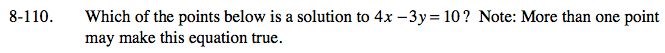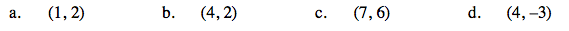### Home > CAAC > Chapter 8 > Lesson 8.3.2 > Problem8-110

8-110.
1. Which of the points below is a solution to 4x − 3y = 10? Note: More than one point may make this equation true. Homework Help ✎

1. (1, 2)

2. (4, 2)

3. (7, 6)

4. (4, −3)Substitute the values for x and y into the equation and evaluate.